Test Description

## 15 Questions MCQ Test Science Olympiad Class 9 | Olympiad Test: Series

Olympiad Test: Series for Class 9 2023 is part of Science Olympiad Class 9 preparation. The Olympiad Test: Series questions and answers have been prepared according to the Class 9 exam syllabus.The Olympiad Test: Series MCQs are made for Class 9 2023 Exam. Find important definitions, questions, notes, meanings, examples, exercises, MCQs and online tests for Olympiad Test: Series below.
 1 Crore+ students have signed up on EduRev. Have you?
Olympiad Test: Series - Question 1

### Direction: Each of following question consists of five figures marked A, B, C, D and E called the Problem Figures followed by five other figures marked 1, 2, 3, 4 and 5 called the Answer Figures. Select a figure from amongst the Answer Figures which will continue the same series as established by the five Problem Figures. Problem Figure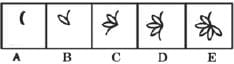Answer Figure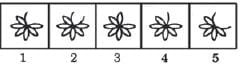Detailed Solution for Olympiad Test: Series - Question 1

Two and three half-leaves are added to the figure alternately. The addition of half-leaves takes place in an ACW direction.

Olympiad Test: Series - Question 2

### Direction: Each of following question consists of five figures marked A, B, C, D and E called the Problem Figures followed by five other figures marked 1, 2, 3, 4 and 5 called the Answer Figures. Select a figure from amongst the Answer Figures which will continue the same series as established by the five Problem Figures. Problem Figure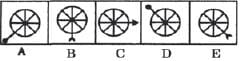Answer Figure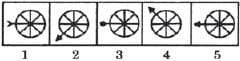Detailed Solution for Olympiad Test: Series - Question 2

The arrow moves one, two, three, four, ...... spaces ACW sequentially. The arrowhead changes in the sequence: circle → arc → triangle → circle → ....

Olympiad Test: Series - Question 3

### Direction: Each of following question consists of five figures marked A, B, C, D and E called the Problem Figures followed by five other figures marked 1, 2, 3, 4 and 5 called the Answer Figures. Select a figure from amongst the Answer Figures which will continue the same series as established by the five Problem Figures. Problem Figure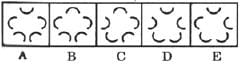Answer Figure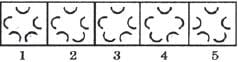Detailed Solution for Olympiad Test: Series - Question 3

One, two, three, one, two, three, ...... arcs get inverted sequentially. This inversion takes place in an ACW direction.

Olympiad Test: Series - Question 4

Direction: Each of following question consists of five figures marked A, B, C, D and E called the Problem Figures followed by five other figures marked 1, 2, 3, 4 and 5 called the Answer Figures. Select a figure from amongst the Answer Figures which will continue the same series as established by the five Problem Figures.
Problem Figure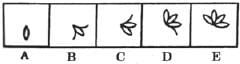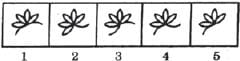Detailed Solution for Olympiad Test: Series - Question 4

In one step, one half-leaf is lost from the CW end and three half-leaves are added at the ACW end. In the next step, one half-leaf is lost from the CW-end and two half-leaves are added at the ACW-end.

Olympiad Test: Series - Question 5

Direction: Each of following question consists of five figures marked A, B, C, D and E called the Problem Figures followed by five other figures marked 1, 2, 3, 4 and 5 called the Answer Figures. Select a figure from amongst the Answer Figures which will continue the same series as established by the five Problem Figures.
Problem Figure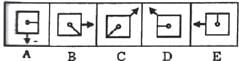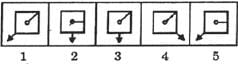Detailed Solution for Olympiad Test: Series - Question 5

The pin rotates 45° CW and 90° CW alternately and moves one space (each space in equal to half-a-side of the square) and two spaces CW alternately. The arrow rotates 90° ACW and 45° ACW alternately and moves two spaces and one space.

Olympiad Test: Series - Question 6

Direction: Each of following question consists of five figures marked A, B, C, D and E called the Problem Figures followed by five other figures marked 1, 2, 3, 4 and 5 called the Answer Figures. Select a figure from amongst the Answer Figures which will continue the same series as established by the five Problem Figures.
Problem Figure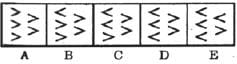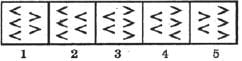Detailed Solution for Olympiad Test: Series - Question 6

In each step, one of the elements gets laterally inverted.

Olympiad Test: Series - Question 7

Direction: Each of following question consists of five figures marked A, B, C, D and E called the Problem Figures followed by five other figures marked 1, 2, 3, 4 and 5 called the Answer Figures. Select a figure from amongst the Answer Figures which will continue the same series as established by the five Problem Figures.
Problem Figure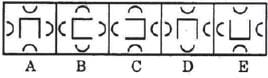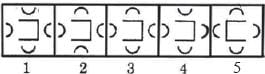Detailed Solution for Olympiad Test: Series - Question 7

Three and two arcs are inverted alternately. The central element rotates 90° ACW and 180° alternately.

Olympiad Test: Series - Question 8

Direction: Each of following question consists of five figures marked A, B, C, D and E called the Problem Figures followed by five other figures marked 1, 2, 3, 4 and 5 called the Answer Figures. Select a figure from amongst the Answer Figures which will continue the same series as established by the five Problem Figures.
Problem Figure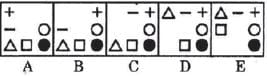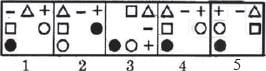Detailed Solution for Olympiad Test: Series - Question 8

In each step, the CW-end element moves to the ACW-end position.

Olympiad Test: Series - Question 9

Direction: Each of following question consists of five figures marked A, B, C, D and E called the Problem Figures followed by five other figures marked 1, 2, 3, 4 and 5 called the Answer Figures. Select a figure from amongst the Answer Figures which will continue the same series as established by the five Problem Figures.
Problem Figure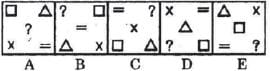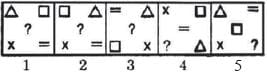Detailed Solution for Olympiad Test: Series - Question 9

In each step, the elements move in the sequence.

Olympiad Test: Series - Question 10

Direction: Each of following question consists of five figures marked A, B, C, D and E called the Problem Figures followed by five other figures marked 1, 2, 3, 4 and 5 called the Answer Figures. Select a figure from amongst the Answer Figures which will continue the same series as established by the five Problem Figures.
Problem Figure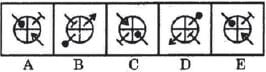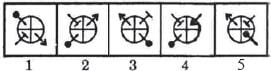Detailed Solution for Olympiad Test: Series - Question 10

All the elements move one space ACW (each space is equal to a quadrant of the circle) and get inverted in each step.

Olympiad Test: Series - Question 11

Direction: Each of following question consists of five figures marked A, B, C, D and E called the Problem Figures followed by five other figures marked 1, 2, 3, 4 and 5 called the Answer Figures. Select a figure from amongst the Answer Figures which will continue the same series as established by the five Problem Figures.
Problem Figure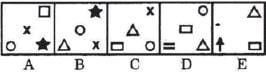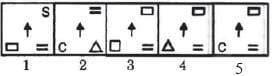Detailed Solution for Olympiad Test: Series - Question 11

In each step, the elements move in the sequence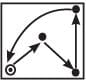and the element that reaches the encircled position gets replaced by a new element.

Olympiad Test: Series - Question 12

Direction: Each of following question consists of five figures marked A, B, C, D and E called the Problem Figures followed by five other figures marked 1, 2, 3, 4 and 5 called the Answer Figures. Select a figure from amongst the Answer Figures which will continue the same series as established by the five Problem Figures.
Problem Figure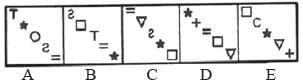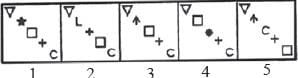Detailed Solution for Olympiad Test: Series - Question 12

In each step, the symbols move in the sequence and the symbol that reaches the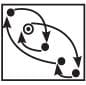encircled position gets replaced by a new one.

Olympiad Test: Series - Question 13

Direction: Each of following question consists of five figures marked A, B, C, D and E called the Problem Figures followed by five other figures marked 1, 2, 3, 4 and 5 called the Answer Figures. Select a figure from amongst the Answer Figures which will continue the same series as established by the five Problem Figures.
Problem Figure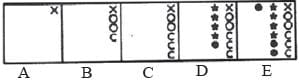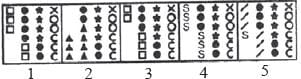Detailed Solution for Olympiad Test: Series - Question 13

The number of symbols added sequentially is 3, 2, 5, 2, 7, 2, ..... These symbols are added to form a sequence of 1, 2, 3, 4, 5, 6 identical symbols.

Olympiad Test: Series - Question 14

Direction: Each of following question consists of five figures marked A, B, C, D and E called the Problem Figures followed by five other figures marked 1, 2, 3, 4 and 5 called the Answer Figures. Select a figure from amongst the Answer Figures which will continue the same series as established by the five Problem Figures.
Problem Figure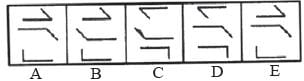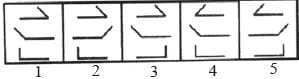Detailed Solution for Olympiad Test: Series - Question 14

In one step, the middle element rotates through 180° and in the next step, the other two elements rotate through 180°. The two steps are repeated alternately.

Olympiad Test: Series - Question 15

Direction: Each of following question consists of five figures marked A, B, C, D and E called the Problem Figures followed by five other figures marked 1, 2, 3, 4 and 5 called the Answer Figures. Select a figure from amongst the Answer Figures which will continue the same series as established by the five Problem Figures.
Problem Figure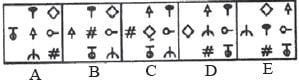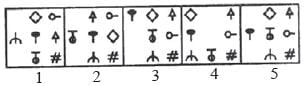Detailed Solution for Olympiad Test: Series - Question 15

In one step, the elements move in the sequence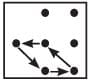and in the next step, the elements move in the sequence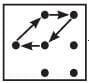. The two steps are repeated alternately.